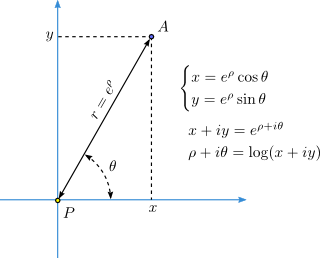### Log-Polar coordinate system applied to Google maps

Inspired by XKCD strip #485: Depth.Log-Polar view of the Earth, centered near the Eiffel Tower, Paris. Click to view full 2k x 4k image.

Coordinate system is a way to assign numeric coordinates to every point of a plane (or space). Cartesian coordinate system is definitely the most used one. Polar coordinate system is probably the second in the popularity ranking, it identifies points by two numbers $$(r,\theta)$$, where $$r$$ is the distance from the point to the pole, and $$\theta$$ is the angle between radius-vector of a point and some fixed direction: polar axis.

Logarithmic-Polar coordinate system (Wikipedia) is the modification of the latter, which instead of distance $$r$$ uses its logarithm: $$\rho=\log r$$. Isn't it just a complication? Not at all. Arguably, log-polar coordinates are more natural than simple polar.Relation between Cartesian $$(x,y)$$, polar $$(r,\theta)$$ and log-polar $$(\rho,\theta)$$ coordinates of the point A.

Cartesian coordinates arise naturally when you need to express translations (movements) of an object in space. To translate a point in some direction, one should simply increment its coordinates by corresponding amount.

Similarly, the log-polar coordinate system is naturally related to the rotation and scale transformations:

• rotation around the pole by angle $$\phi$$ corresponds to increment of the angular coordinate: $$\theta_1 = \theta + \phi$$,
• uniform scaling with factor $$k$$ is equivalent to increment of the logarithmic length: $$\rho_1 = \rho + \log k$$.

But there is more. Mapping from log-polar coordinates to Cartesian is conformal. This can be easily seen using complex plane: If some point A has Cartesian coordinates $$(x,y)$$ and log-polar coordinates $$(\rho, \theta)$$, then according to Euler's formula:
$$x+iy = e^{\rho+i\theta} ,$$ $$\rho+i\theta = \log(x+iy),$$
where $$i$$ is complex unit. Since logarithm and exponent are analytic functions, this mapping is conformal.

Let's apply log-polar to Cartesian coordinate transform to an image, mapping log-distance $$\rho$$ to the vertical Cartesian axis and angle $$\theta$$ to horizontal. Conformity of this mapping preserves angles between lines. Straight lines will become curves, but they will intersect at the same angles as in the original image. And the smaller the fragment of the image, the less distorted it would appear.

Interesting property of this mapping is that it shows both small and large scale features on the same picture, smohly connected. If $$\rho=\log r$$ is mapped to the vertical coordinate axis, then small objects near the center and big objects far from the center will appear the same size, just one higher than another. Thanks to quick growth of the exponent function, it is possible to show both atomic nuclei and planets on the same chart (XKCD #485).

Enough words, see the images. They are clickable, hyperlink leads to the full-size gallery. Beware of traffic.Log-Polar satellite view of the Earth, center is placed near the Eiffel Tower. Click to view full 2k x 4k image.

The above picture shows the whole hemisphere of the Earth, originally in orthogonal projection. Log-polar to Cartesian coordinate mapping makes scale uniformly grow from bottom to top. You can see both singe pedestrians at the bottom and whole continents (yellow blob at the top is Africa) in the upper part.

## Software used

Python scripts used to construct these images are available in the Github repository:

under the permissive MIT license. To run these scripts, you'll need Python 3.3+ and Pillow. For the time being, the scripts are not well organized. Run the auto_glue.py --help to see help on options.

The script uses Google Maps static API to download multiple images of the same place with different zoom levels. Each piece of the map is first converted from Mercator to orthogonal projection. Then orthogonal projection is transformed from log-polar to Cartesian coordinate system, producing one horizontal band. All bands are then glued together into the single image.